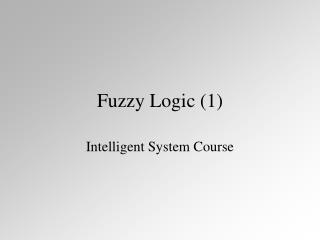DownloadDownload PresentationFuzzy Logic (1)

# Fuzzy Logic (1)

Télécharger la présentation## Fuzzy Logic (1)

- - - - - - - - - - - - - - - - - - - - - - - - - - - E N D - - - - - - - - - - - - - - - - - - - - - - - - - - -
##### Presentation Transcript

1. Fuzzy Logic (1) Intelligent System Course

2. A A A A A A A O O O O O O O Group of apple Group of orange Apples, oranges or in between?

4. A A A A A A A O O O O O O O Thinking in Fuzzy Fuzzy group of apples Fuzzy group of oranges

5. Crisp vs Fuzzy • Crisp sets handle only 0s and 1s • Fuzzy sets handle all values between 0 and 1 Crisp No Yes Fuzzy No Slightly Mostly Yes

6. Fuzzy Set Notation Continuous Discrete

7. B(x) 0 1 2 3 x Continuous Fuzzy Membership Function • The set, B, of numbers near to two is

8. B(x) 0 1 2 3 x Discrete Fuzzy Membership Function The set, B, of numbers near to two is

9. B(x) 1 0 1 2 3 x A(x) 0 1 2 3 x Example Example Fuzzy Sets to Aggregate... A = { x | x is near an integer} B = { x | x is close to 2}

10. Fuzzy Union • Fuzzy Union (logic “or”) • Meets crisp boundary conditions • Commutative • Associative • Idempotent

11. A+B (x) 0 1 2 3 x Fuzzy Union - example A OR B = A+B ={ x | (x is near an integer) OR (x is close to 2)} = MAX [A(x), B(x)]

12. Fuzzy Intersection • Fuzzy Intersection (logic “and”) • Meets crisp boundary conditions • Commutative • Associative • Idempotent

13. AB(x) 0 1 2 3 x Fuzzy Intersection - example A AND B = A·B ={ x | (x is near an integer) AND (x is close to 2)} = MIN [A(x), B(x)]

14. Fuzzy Complement The complement of a fuzzy set has a membership function... • Meets crisp boundary conditions • Two Negatives Should Make a Positive • Law of Excluded Middle: NO! • Law of Contradiction: NO!

15. 1 0 1 2 3 x Fuzzy Complement - example complement of A ={ x | x is notnear an integer}

16. Fuzzy Associativity (1) Min-Max fuzzy logic has intersection distributive over union... since min[ A,max(B,C) ]=min[ max(A,B), max(A,C) ]

17. Fuzzy Associativity (2) Min-Max fuzzy logic has union distributive over intersection... since max[ A,min(B,C) ]= max[ min(A,B), min(A,C) ]

18. Fuzzy and DeMorgan’s Law (1) Min-Max fuzzy logic obeys DeMorgan’s Law #1... since 1 - min(B,C)= max[ (1-B), (1-C)]

19. Fuzzy and DeMorgan’s Law (2) Min-Max fuzzy logic obeys DeMorgans Law #2... since 1 - max(B,C)= min[(1-B), (1-C)]

20. Fuzzy and Excluded Middle Min-Max fuzzy logic fails The Law of Excluded Middle. since min(A,1-A)  0 Thus, (the set of numbers close to 2) AND (the set of numbers notclose to 2)  null set

21. Fuzzy and the Law of Contradiction Min-Max fuzzy logic fails theThe Law of Contradiction. since max(A,1-A)  1 Thus, (the set of numbers close to 2) OR (the set of numbers notclose to 2)  universal set

22. Cartesian Product • The intersection and union operations can also be used to assign memberships on the Cartesian product of two sets. • Consider, as an example, the fuzzy membership of a set, G, of liquids that taste good and the set, LA, of cities close to Los Angeles

23. LosAngeles(0.0) Chicago (0.5) New York (0.8) London(0.9) Swamp Water (0.0) 0.00 0.00 0.00 0.00 Radish Juice (0.5) 0.00 0.50 0.50 0.50 Grape Juice (0.9) 0.00 0.50 0.80 0.90 Cartesian Product • We form the set... • E = G ·LA • = liquids that taste goodAND cities that • are close to LA • The following table results...